Home » CNN vs. RNN vs. ANN – Analyzing 3 Types of Neural Networks in Deep Learning

# CNN vs. RNN vs. ANN – Analyzing 3 Types of Neural Networks in Deep Learning

## Overview

• Check out 3 different types of neural networks in deep learning
• Understand when to use which type of neural network for solving a deep learning problem
• We will also compare these different types of neural networks in an easy-to-read tabular format!

## Why Deep Learning?

It’s a pertinent question. There is no shortage of machine learning algorithms so why should a data scientist gravitate towards deep learning algorithms? What do neural networks offer that traditional machine learning algorithms don’t?

Another common question I see floating around – neural networks require a ton of computing power, so is it really worth using them? While that question is laced with nuance, here’s the short answer – yes!

The different types of neural networks in deep learning, such as convolutional neural networks (CNN), recurrent neural networks (RNN), artificial neural networks (ANN), etc. are changing the way we interact with the world. These different types of neural networks are at the core of the deep learning revolution, powering applications like unmanned aerial vehicles, self-driving cars, speech recognition, etc.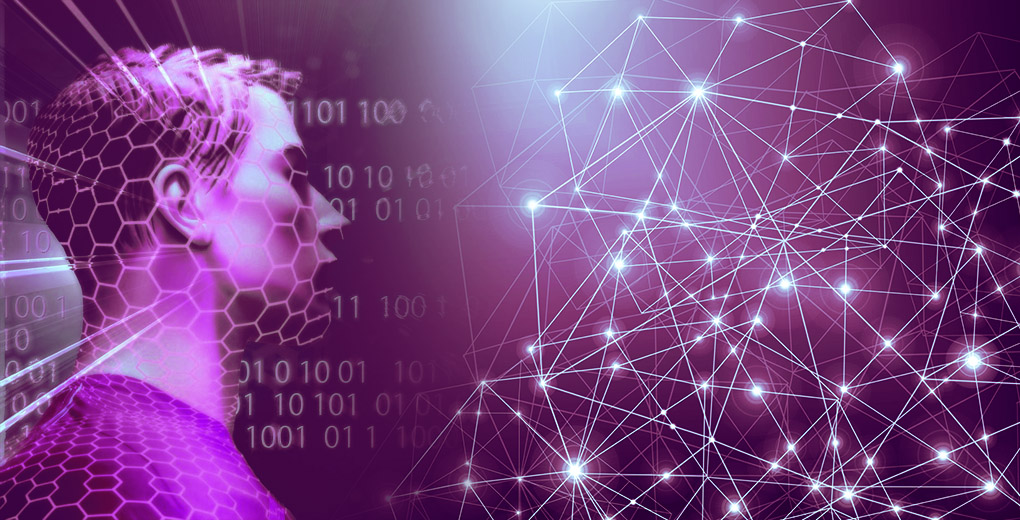It’s natural to wonder – can’t machine learning algorithms do the same? Well, here are two key reasons why researchers and experts tend to prefer Deep Learning over Machine Learning:

• Decision Boundary
• Feature Engineering

Curious? Good – let me explain.

### 1. Machine Learning vs. Deep Learning: Decision Boundary

Every Machine Learning algorithm learns the mapping from an input to output. In case of parametric models, the algorithm learns a function with a few sets of weights:

Input -> f(w1,w2…..wn) -> Output

In the case of classification problems,  the algorithm learns the function that separates 2 classes – this is known as a Decision boundary. A decision boundary helps us in determining whether a given data point belongs to a positive class or a negative class.

For example, in the case of logistic regression, the learning function is a Sigmoid function that tries to separate the 2 classes: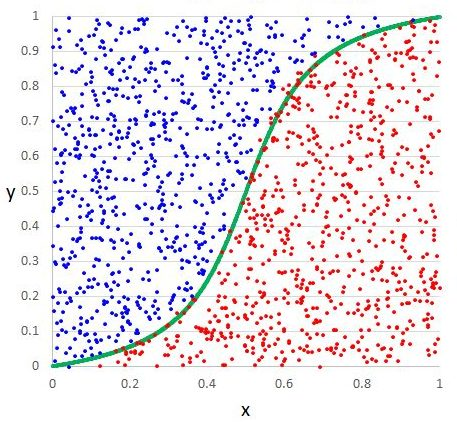Decision boundary of logistic regression

As you can see here, the logistic regression algorithm learns the linear decision boundary. It cannot learn decision boundaries for nonlinear data like this one: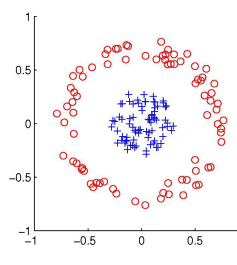Nonlinear data

Similarly, every Machine Learning algorithm is not capable of learning all the functions. This limits the problems these algorithms can solve that involve a complex relationship.

### 2. Machine Learning vs. Deep Learning: Feature Engineering

Feature engineering is a key step in the model building process. It is a two-step process:

1. Feature extraction
2. Feature selection

In feature extraction, we extract all the required features for our problem statement and in feature selection, we select the important features that improve the performance of our machine learning or deep learning model.

Consider an image classification problem. Extracting features manually from an image needs strong knowledge of the subject as well as the domain. It is an extremely time-consuming process. Thanks to Deep Learning, we can automate the process of Feature Engineering!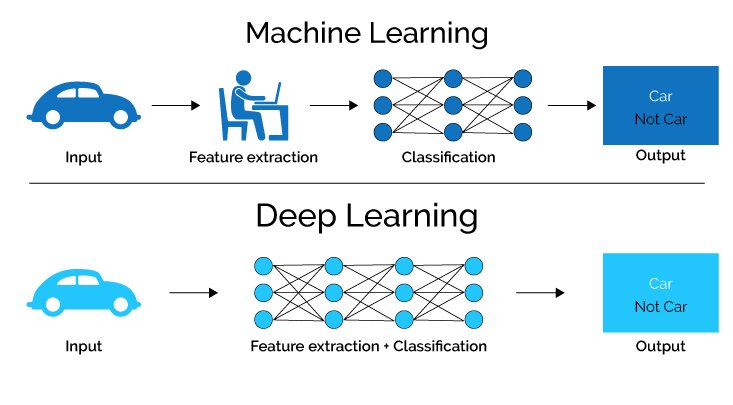Comparison between Machine Learning & Deep Learning

Now that we understand the importance of deep learning and why it transcends traditional machine learning algorithms, let’s get into the crux of this article. We will discuss the different types of neural networks that you will work with to solve deep learning problems.

If you are just getting started with Machine Learning and Deep Learning, here is a course to assist you in your journey:

## Different types of Neural Networks in Deep Learning

This article focuses on three important types of neural networks that form the basis for most pre-trained models in deep learning:

• Artificial Neural Networks (ANN)
• Convolution Neural Networks (CNN)
• Recurrent Neural Networks (RNN)

Let’s discuss each neural network in detail.

## Artificial Neural Network (ANN) – What is a ANN and why should you use it?

A single perceptron (or neuron) can be imagined as a Logistic Regression. Artificial Neural Network, or ANN, is a group of multiple perceptrons/ neurons at each layer. ANN is also known as a Feed-Forward Neural network because inputs are processed only in the forward direction: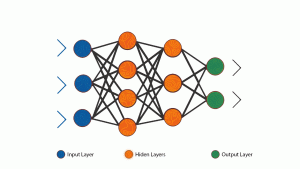ANN

As you can see here, ANN consists of 3 layers – Input, Hidden and Output. The input layer accepts the inputs, the hidden layer processes the inputs, and the output layer produces the result. Essentially, each layer tries to learn certain weights.

If you want to explore more about how ANN works, I recommend going through the below article:

ANN can be used to solve problems related to:

• Tabular data
• Image data
• Text data

### Advantages of Artificial Neural Network (ANN)

Artificial Neural Network is capable of learning any nonlinear function. Hence, these networks are popularly known as Universal Function Approximators. ANNs have the capacity to learn weights that map any input to the output.

One of the main reasons behind universal approximation is the activation function. Activation functions introduce nonlinear properties to the network. This helps the network learn any complex relationship between input and output.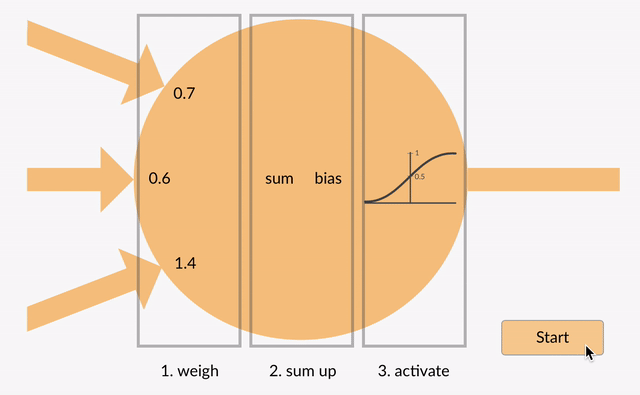Perceptron

As you can see here, the output at each neuron is the activation of a weighted sum of inputs. But wait –  what happens if there is no activation function? The network only learns the linear function and can never learn complex relationships. That’s why:

An activation function is a powerhouse of ANN!

### Challenges with Artificial Neural Network (ANN)

• While solving an image classification problem using ANN, the first step is to convert a 2-dimensional image into a 1-dimensional vector prior to training the model. This has two drawbacks:
• The number of trainable parameters increases drastically with an increase in the size of the image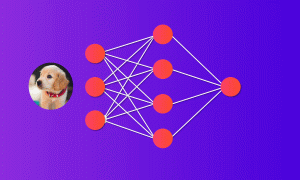ANN: Image classification

In the above scenario, if the size of the image is 224*224, then the number of trainable parameters at the first hidden layer with just 4 neurons is 602,112. That’s huge!

•  ANN loses the spatial features of an image. Spatial features refer to the arrangement of the pixels in an image. I will touch upon this in detail in the following sections
• One common problem in all these neural networks is the Vanishing and Exploding Gradient. This problem is associated with the backpropagation algorithm. The weights of a neural network are updated through this backpropagation algorithm by finding the gradients: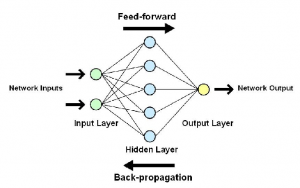Backward Propagation

So, in the case of a very deep neural network (network with a large number of hidden layers), the gradient vanishes or explodes as it propagates backward which leads to vanishing and exploding gradient.

• ANN cannot capture sequential information in the input data which is required for dealing with sequence data

Now, let us see how to overcome the limitations of MLP using two different architectures – Recurrent Neural Networks (RNN) and Convolution Neural Networks (CNN).

## Recurrent Neural Network (RNN) – What is an RNN and why should you use it?

Let us first try to understand the difference between an RNN and an ANN from the architecture perspective:

A looping constraint on the hidden layer of ANN turns to RNN.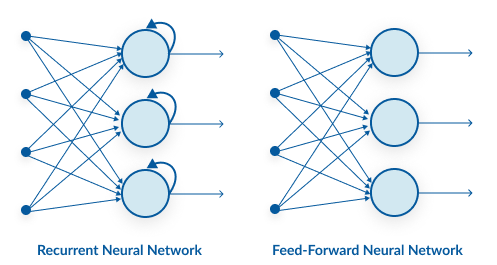As you can see here, RNN has a recurrent connection on the hidden state. This looping constraint ensures that sequential information is captured in the input data.

You should go through the below tutorial to learn more about how RNNs work under the hood (and how to build one in Python):

We can use recurrent neural networks to solve the problems related to:

• Time Series data
• Text data
• Audio data

### Advantages of Recurrent Neural Network (RNN)

• RNN captures the sequential information present in the input data i.e. dependency between the words in the text while making predictions: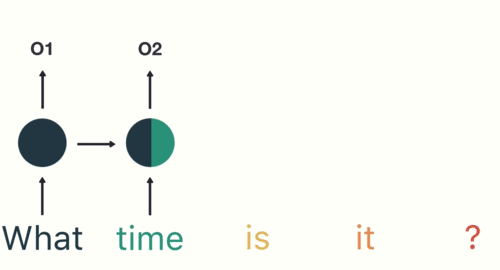Many2Many Seq2Seq model

As you can see here, the output (o1, o2, o3, o4)  at each time step depends not only on the current word but also on the previous words.

• RNNs share the parameters across different time steps. This is popularly known as Parameter Sharing. This results in fewer parameters to train and decreases the computational cost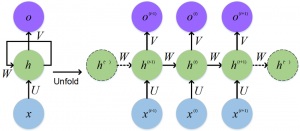Unrolled RNN

As shown in the above figure, 3 weight matrices – U, W, V, are the weight matrices that are shared across all the time steps.

### Challenges with Recurrent Neural Networks (RNN)

Deep RNNs (RNNs with a large number of time steps) also suffer from the vanishing and exploding gradient problem which is a common problem in all the different types of neural networks.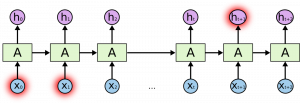As you can see here, the gradient computed at the last time step vanishes as it reaches the initial time step.

## Convolution Neural Network (CNN) – What is a CNN and Why Should you use it?

Convolutional neural networks (CNN) are all the rage in the deep learning community right now. These CNN models are being used across different applications and domains, and they’re especially prevalent in image and video processing projects.

The building blocks of CNNs are filters a.k.a. kernels. Kernels are used to extract the relevant features from the input using the convolution operation. Let’s try to grasp the importance of filters using images as input data. Convolving an image with filters results in a feature map: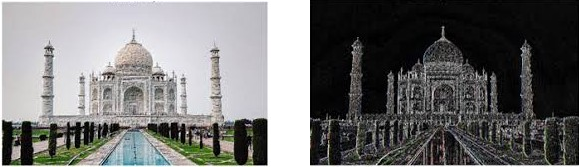Output of Convolution

Want to explore more about Convolution Neural Networks? I recommend going through the below tutorial:

You can also enrol in this free course on CNN to learn more about them: Convolutional Neural Networks from Scratch

Though convolutional neural networks were introduced to solve problems related to image data, they perform impressively on sequential inputs as well.

### Advantages of Convolution Neural Network (CNN)

• CNN learns the filters automatically without mentioning it explicitly. These filters help in extracting the right and relevant features from the input data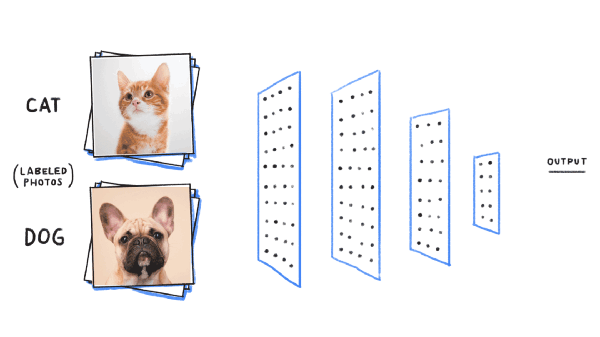CNN – Image Classification

• CNN captures the spatial features from an image. Spatial features refer to the arrangement of pixels and the relationship between them in an image. They help us in identifying the object accurately, the location of an object, as well as its relation with other objects in an imageIn the above image, we can easily identify that its a human’s face by looking at specific features like eyes, nose, mouth and so on. We can also see how these specific features are arranged in an image. That’s exactly what CNNs are capable of capturing.

• CNN also follows the concept of parameter sharing. A single filter is applied across different parts of an input to produce a feature map: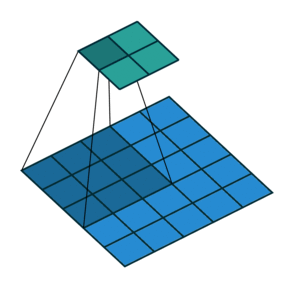Convolving image with a filter

Notice that the 2*2 feature map is produced by sliding the same 3*3 filter across different parts of an image.

### Comparing the Different Types of Neural Networks (MLP(ANN)  vs. RNN vs. CNN)

Here, I have summarized some of the differences among different types of neural networks: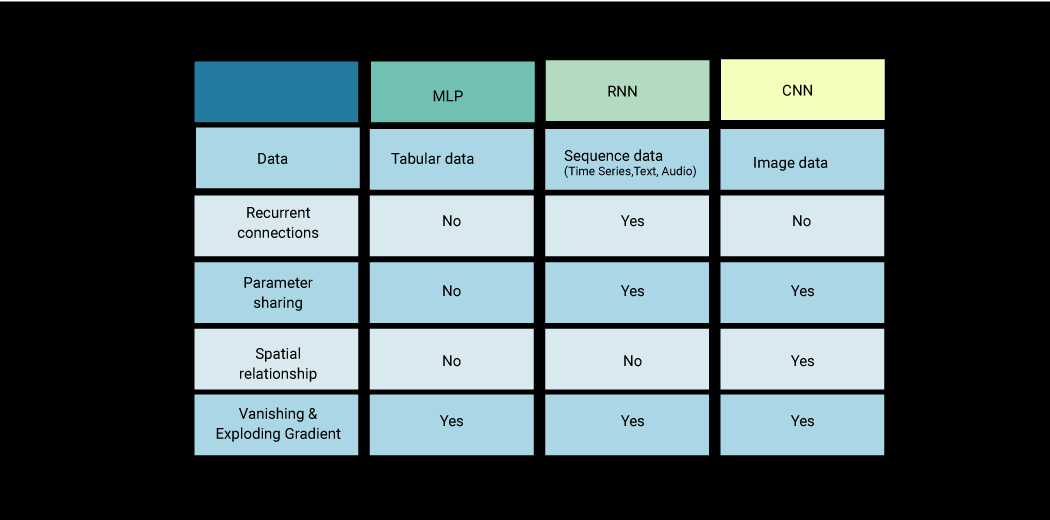## End Notes

In this article, I have discussed the importance of deep learning and the differences among different types of neural networks. I strongly believe that knowledge sharing is the ultimate form of learning. I am looking forward to hearing a few more differences!

You can also read this article on our Mobile APP•Chandrasekhar says:

good one. Refreshing the concepts in quick time . 🙂 🙂 Thanks !

•Prabhanjan Gururaj says:

That is a good one Aravind. Helpful. Thanks

•Vlad says:

Wow, great visualization! Thank you!

•Annapurna says:

This is good one very knowledge